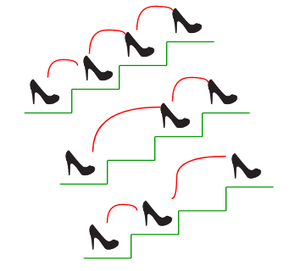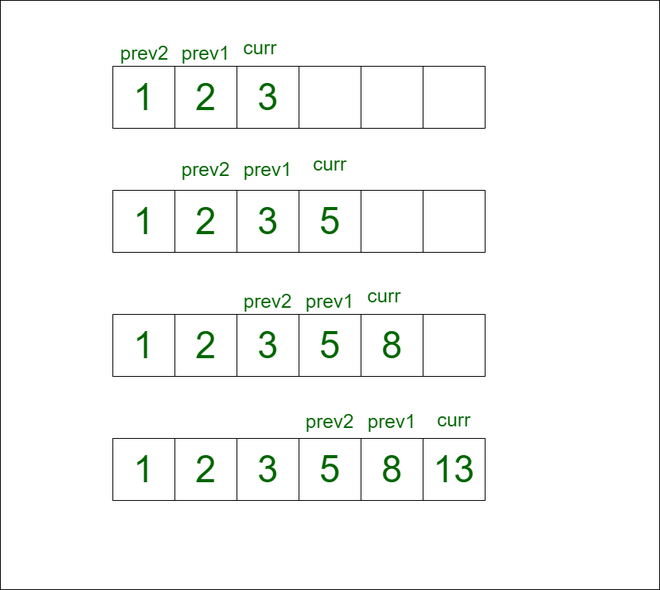GeeksforGeeks App
Open AppBrowser
Continue

# Count ways to reach the Nth stair | Set-2

There are N stairs, a person standing at the bottom wants to reach the top. The person can climb either 1 stair or 2 stairs at a time. Count the number of ways, the person can reach the top.Nth-stairs

Examples:

Input: N = 1
Output: 1
Explanation: There is only one way to climb 1st stair

Input: N= 2
Output: 2
Explanation: There are two ways and the sequence to climb to Nth stair are: (1, 2), (2)

Input: N = 4
Output: 5
Explanation: There are five possible ways and the sequence to climb to Nth stair are:
(1, 2, 3, 4), (1, 2, 4), (2, 3, 4), (1, 3, 4), (2, 4)

The dynamic programming and sliding window and several other approaches are discussed in Set-1 of this problem. But this approach will have a better overall auxiliary space.

Approach: This problem can be solved with better space complexity based on the following observation:

If observed closely, the value of current state ( i ) depends on two states: the value of the previous state (i – 1) and the value of (i – 2) state.
Instead of using extra space only two variables can be used to store the values of the above-mentioned two states.
As one can either climb one step or two step at a time, so ith state value is the summation of the values of (i – 1)th and (i – 2)th state.

Follow the illustration given below for a better understanding.

Illustration:

Initially, There are one ways to reach 1st stair and the sequence to climb to 1st stair is: (1)
There are two ways to reach 2nd stair and the sequence to climb to 2nd stair are: (1, 2), (2)

For 3rd stair:
=> Number of ways to reach 3rd stair = prev1 + prev2. i.e. curr = 2 + 1 = 3.
=> prev2 = prev1 = 2. prev1 = curr = 3

For 4th stair:
=> Number of ways to reach 3rd stair = prev1 + prev2. i.e. curr = 3 + 2 = 5.
=> prev2 = prev1 = 3. prev1 = curr = 5

For 5th stair:
=> Number of ways to reach 3rd stair = prev1 + prev2. i.e. curr = 5 + 3 = 8.
=> prev2 = prev1 = 5. prev1 = curr = 8

For 6th stair:
=> Number of ways to reach 3rd stair = prev1 + prev2. i.e. curr = 8 + 5 = 13.
=> prev2 = prev1 = 8. prev1 = curr = 13

Below is the image representation of each step of above explanation:Follow the steps mentioned below to solve the problem:

• Declare two variables (say, prev1 and prev2) to store the previous two states for any current state and initialize them with the number of ways to reach the 1st and 2nd stair.
• Iterate from 3rd stair to Nth stair:
• Calculate the number of ways to reach the current stair as shown in the above observation.
• Now update the prev1 and prev2 variables as per the observation.
• Return the value for the Nth stair obtained as the result.

Below is the implementation of the above approach:

## C++

 `// C++ code to implement the approach` `#include ``using` `namespace` `std;` `// Function to find minimum number of steps``int` `minSteps(``int` `N)``{``    ``// Can make 1 jump only``    ``if` `(N == 1)``        ``return` `1;` `    ``// Can jump like {2} and {1 + 1}``    ``// so two ways for n == 2;``    ``if` `(N == 2)``        ``return` `2;` `    ``int` `curr;``    ``int` `prev2 = 1;``    ``int` `prev1 = 2;` `    ``// Iterate from 3rd stair to nth stair``    ``for` `(``int` `i = 3; i <= N; i++) {``        ``curr = prev1 + prev2;``        ``prev2 = prev1;``        ``prev1 = curr;``    ``}``    ``return` `curr;``}` `// Driver code``int` `main()``{``    ``int` `N = 6;` `    ``// Function call``    ``cout << minSteps(N);``    ``return` `0;``}`

## Java

 `// Java code to implement the approach``import` `java.util.*;` `class` `GFG {` `  ``// Function to find minimum number of steps``  ``static` `int` `minSteps(``int` `N)``  ``{``    ``// Can make 1 jump only``    ``if` `(N == ``1``)``      ``return` `1``;` `    ``// Can jump like {2} and {1 + 1}``    ``// so two ways for n == 2;``    ``if` `(N == ``2``)``      ``return` `2``;` `    ``int` `curr = ``0``;``    ``int` `prev2 = ``1``;``    ``int` `prev1 = ``2``;` `    ``// Iterate from 3rd stair to nth stair``    ``for` `(``int` `i = ``3``; i <= N; i++) {``      ``curr = prev1 + prev2;``      ``prev2 = prev1;``      ``prev1 = curr;``    ``}``    ``return` `curr;``  ``}` `  ``// Driver code``  ``public` `static` `void` `main (String[] args) {``    ``int` `N = ``6``;` `    ``// Function call``    ``System.out.print(minSteps(N));``  ``}``}` `// This code is contributed by hrithikgarg03188.`

## Python3

 `# Python3 code to implement the approach` `# Function to find the minimum number of steps``def` `minSteps(N):` `    ``# Can make 1 jump only``    ``if` `N ``=``=` `1``:``        ``return` `1` `    ``# Can jump like {2} and {1 + 1}``    ``# so two ways for n == 2``    ``if` `N ``=``=` `2``:``        ``return` `2` `    ``prev1, prev2 ``=` `2``, ``1` `    ``# iterate from 3rd stair to nth star``    ``for` `i ``in` `range``(``3``, N ``+` `1``):``        ``curr ``=` `prev1 ``+` `prev2``        ``prev2 ``=` `prev1``        ``prev1 ``=` `curr` `    ``return` `curr` `# Driver Code``N ``=` `6` `# Function call``print``(minSteps(N))` `# This code is contributed by phasing17`

## C#

 `// C# code to implement the above approach``using` `System;` `class` `GFG {` `  ``// Function to find minimum number of steps``  ``static` `int` `minSteps(``int` `N)``  ``{``    ``// Can make 1 jump only``    ``if` `(N == 1)``      ``return` `1;` `    ``// Can jump like {2} and {1 + 1}``    ``// so two ways for n == 2;``    ``if` `(N == 2)``      ``return` `2;` `    ``int` `curr = 0;``    ``int` `prev2 = 1;``    ``int` `prev1 = 2;` `    ``// Iterate from 3rd stair to nth stair``    ``for` `(``int` `i = 3; i <= N; i++) {``      ``curr = prev1 + prev2;``      ``prev2 = prev1;``      ``prev1 = curr;``    ``}``    ``return` `curr;``  ``}` `  ``// Driver code``  ``public` `static` `void` `Main()``  ``{``    ``int` `N = 6;` `    ``// Function call``    ``Console.Write(minSteps(N));``  ``}``}` `// This code is contributed by sanjoy_62.`

## Javascript

 ``

Output

`13`

Time Complexity: O(N)
Auxiliary Space: O(1)

My Personal Notes arrow_drop_up